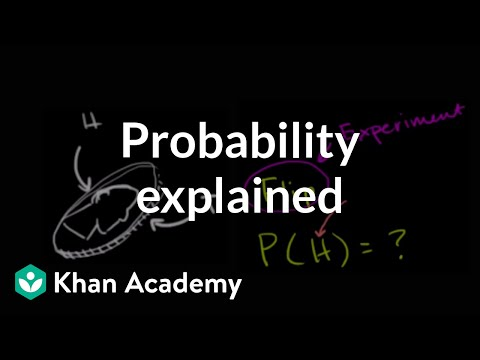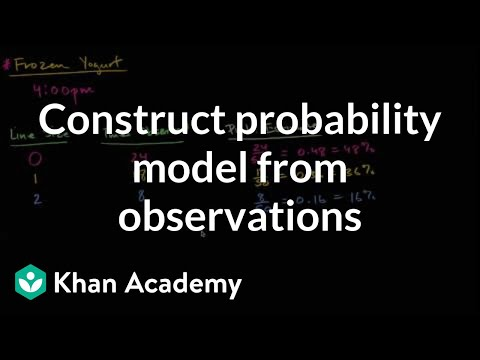Video

# Intuitive sense of probabilities (Full video)

Description: Think about what probabilities really mean. What does a probability of 0 mean? How about 1? Now let's say we ask ourselves what is the probability of rolling a number that is less than or equal to two? So there is a 2/6 probability of rolling a number less than or equal to two. What is the probability of rolling a number greater than or equal to three?

### Other videos you might be interested in### Intro to theoretical probability (Full video)